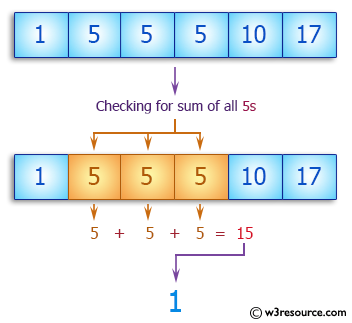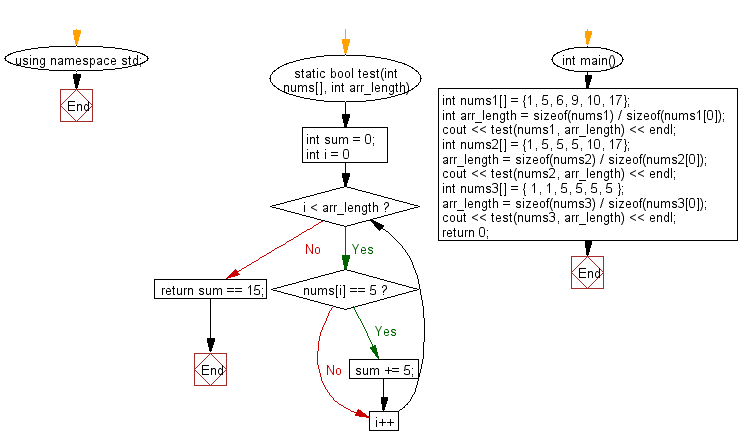﻿ C++ : Check if the sum of all 5 exactly 15 in an array# C++ Exercises: Check if the sum of all 5' in the array exactly 15 in a given array of integers

## C++ Basic Algorithm: Exercise-104 with Solution

Write a C++ program to check if the sum of all 5' in the array exactly 15 in a given array of integers.

Sample Solution:

C++ Code :

``````#include <iostream>
using namespace std;

static bool test(int nums[], int arr_length)
{
int sum = 0;

for (int i = 0; i < arr_length; i++)
{
if (nums[i] == 5)
sum += 5;
}

return sum == 15;
}

int main()
{
int nums1[] = {1, 5, 6, 9, 10, 17};
int arr_length = sizeof(nums1) / sizeof(nums1);
cout << test(nums1, arr_length) << endl;
int nums2[] = {1, 5, 5, 5, 10, 17};
arr_length = sizeof(nums2) / sizeof(nums2);
cout << test(nums2, arr_length) << endl;
int nums3[] = { 1, 1, 5, 5, 5, 5 };
arr_length = sizeof(nums3) / sizeof(nums3);
cout << test(nums3, arr_length) << endl;
return 0;
}
``````

Sample Output:

```0
1
0
```

Pictorial Presentation:Flowchart:C++ Code Editor:

Contribute your code and comments through Disqus.

What is the difficulty level of this exercise?

﻿